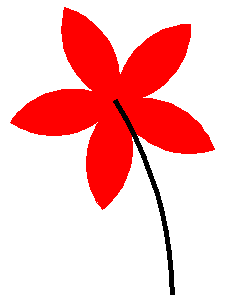deutsch     english    français     Print

## 2.4 FUNCTIONS### INTRODUCTION

 In a larger picture, you may want to use figures such as triangles and squares repeatedly. However, the turtle itself does not know what a triangle or a square is. Therefore you have to explain to the turtle how to draw the figures each time with a complete program code. Is this possible in an easier way? It is! You can teach the turtle new commands, for example how to draw a square or a triangle. Then you simply have to tell the turtle that it should execute such a command, namely draw a square or a triangle. In order to define a new command, you can choose any given identifier, for example square, and then write def square(): After that, you then write down all of the instructions belonging to the new command. In order for the computer to know what is part of the new command, the instructions must be indented. PROGRAMMING CONCEPTS: Modular programming, function definition, function call

###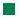In this program you will use def to define the new command square(). Afterwards, the turtle will know how to draw a square, however, it will not have drawn one yet. Using the command square() the turtle draws a square at its current position with a side length of 100. In our example there is a red, a blue, and a green square.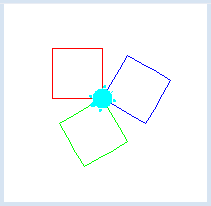```from gturtle import *

def square():
repeat 4:
forward(100)
left(90)

makeTurtle()
setPenColor("red")
square()
right(120)
setPenColor("blue")
square()
right(120)
setPenColor("green")
square()
```
Highlight program code (Ctrl+C copy, Ctrl+V paste)

### MEMO

 You can define a new command using def identifier(): Choose a name that reflects the activity of the command. All instructions that belong to the new command must be indented ```def identifier(): instuctions ``` Do not forget to put brackets and the colon after the identifier! In Python you also call new commands functions. When you use the function square() one could also say that the function is "called". We should get used to placing the function definitions in the program header, since they have to be defined before they are called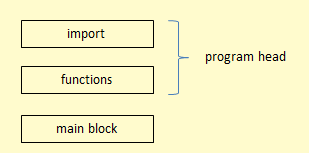### EXERCISES

1.

 Define a command hexagon() with which you can draw a hexagon, then use this command to draw the adjacent figure.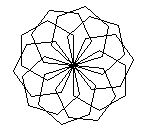2a.

 Define a command that draws a square standing on one of its corners, then use this command to draw the adjacent figure.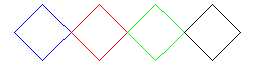2b*.

 You can draw filled squares by using the commands startPath() and fillPath().3a.

 In this task, you will experience how to solve a problem step by step using functions [more... It is a fundamental programming concept, called procedural programming]. Define a function arc() that tells the turtle to draw an arc and rotate a total of 90 degrees to the right. You can set the maximum value of the turtle's speed with speed(-1).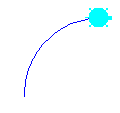3b.

 Add the function petal() to the program, which will draw two arcs. At the end, the turtle should be back in the original starting direction.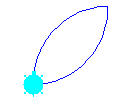3c.

 Add another command to the program so that the petal() is drawn as a filled red leaf (without a visible border line).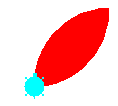3d.

 Extend the program with the function flower(), which draws a five-petalled flower. To make the turtle draw the flower even faster, use the function hideTurtle() to make the turtle invisible.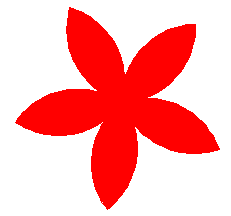3e*.

 Add a stem to the flower.